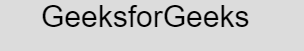# p5.js textSize() Function

The textSize() function in p5.js is used to set or return the font size of text. This function is used in all subsequent calls to the text() function.

Syntax:

`textSize(size)`

or

`textSize()`

Parameters: This function accepts single parameter sizewhich stores the size of the text in terms of pixels.

Below programs illustrate the textSize() function in p5.js:

Example 1: This example uses textSize() function to set the font size of text.

 `function` `setup() { ` `     `  `    ``// Create Canvas of given size ` `    ``createCanvas(380, 170); ` `} ` ` `  `function` `draw() { ` `     `  `    ``let string = ``"GeeksforGeeks"``; ` `     `  `    ``// Set the background color ` `    ``background(220); ` `     `  `    ``// Set the text size ` `    ``textSize(30); ` `     `  `    ``// Set the text  ` `    ``text(string, 100, 30); ` `} `

Output:Example 2: This example uses textSize() function to return the font size of text.

 `function` `setup() { ` `     `  `    ``// Create Canvas of given size ` `    ``createCanvas(380, 170); ` `} ` ` `  `function` `draw() { ` `     `  `    ``let string = ``"GeeksforGeeks"``; ` `     `  `    ``// Set the background color ` `    ``background(220); ` `     `  `    ``// Set the text size ` `    ``textSize(16); ` `     `  `    ``// store the size of text ` `    ``var` `u = textSize(); ` `     `  `    ``// Set the stroke color ` `    ``stroke(255, 204, 0); ` ` `  `    ``// Display result ` `    ``text(``"Value of Text Size is : "` `            ``+ u, 50, 30); ` `} `

Output:Reference: https://p5js.org/reference/#/p5/textSize

Whether you're preparing for your first job interview or aiming to upskill in this ever-evolving tech landscape, GeeksforGeeks Courses are your key to success. We provide top-quality content at affordable prices, all geared towards accelerating your growth in a time-bound manner. Join the millions we've already empowered, and we're here to do the same for you. Don't miss out - check it out now!

Previous
Next